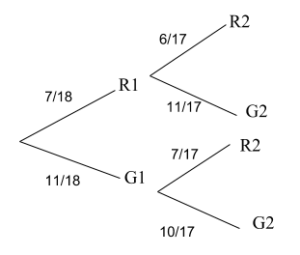# Bayes Theorem

Contents

## Summary

• Bayes theorem is basically defined as calculating the given probability when we know certain other probabilities.
• Bayes theorem can be written as:$P(\frac { B }{ A } )\quad =\quad \frac { P(B)\quad .\quad P(\frac { A }{ B } ) }{ P(\frac { B }{ A } )P(A)\quad +\quad P(B)\quad .\quad P(\frac { A }{ B } ) }$

We have already studied conditional probability in the article ”Probability”.

Let’s recall this before we move on to Bayes theorem.

Conditional probability is when the probability of one event, given that the second event has already occured. It is defined as:$P(\frac { A }{ B } )\quad =\quad \frac { P(A\cap B) }{ P(B) } \quad \quad AND\quad \quad P(\frac { B }{ A } )\quad =\quad \frac { P(B\cap A) }{ P(A) }$

In order to find$P(A\cap B)$ we use the bayes theorem which is defined as calculating the given probability when we know certain other probabilities. It’s formula is:$P(A\cap B)\quad =\quad P(B)\quad .\quad P(\frac { A }{ B } )$$P(B\cap A)\quad =\quad P(\frac { B }{ A } )P(A)$

To simplify, it can be written as:$P(\frac { A }{ B } )\quad =\quad \frac { P(\frac { B }{ A } )P(A) }{ P(B) }$

Now, that we have defined the bayes formula, in order to plug in the values, we require the total probability, which can be written as:$P(A)\quad =\quad \left[ P(\frac { B }{ A } )P(A) \right] \quad +\quad \left[ P(B)\quad .\quad P(\frac { A }{ B } ) \right] \quad$

It can also be written as:$P(A)\quad =\quad \sum _{ i=1 }^{ n }{ P(\frac { A }{ B } ) } P(B)$

Thus the bayes theorem can finally be written as:$P(\frac { B }{ A } )\quad =\quad \frac { P(B)\quad .\quad P(\frac { A }{ B } ) }{ P(\frac { B }{ A } )P(A)\quad +\quad P(B)\quad .\quad P(\frac { A }{ B } ) }$

In a more simplified form it can be written as:$P(\frac { B }{ A } )\quad =\quad \frac { P(B)\quad .\quad P(\frac { A }{ B } ) }{ \sum _{ i=1 }^{ n }{ P(\frac { A }{ B } ) } P(B) }$

#### Example #1

Q. There is a bag of balls which has 7 red balls and 11 green balls.

a) Draw a tree diagram that shows the following information:
If two balls are drawn one after the other, without replacement.b) Find the probability$P({ R }_{ 1 }\cap { G }_{ 2 })\quad =\quad P({ R }_{ 1 })\quad \times \quad P(\frac { { G }_{ 2 } }{ { R }_{ 1 } } )$$\frac { 7 }{ 18 } \quad \times \quad \frac { 11 }{ 17 }$

c) P(Different coloured ball)$P({ R }_{ 1 }\cap { G }_{ 2 })\quad +\quad P({ G }_{ 1 }\cap { R }_{ 2 })$$\frac { 7 }{ 18 } \quad \times \quad \frac { 11 }{ 17 } \quad +\quad \frac { 11 }{ 18 } \quad \times \quad \frac { 7 }{ 17 }$

d) Find the probability that the second ball is green.$P({ R }_{ 1 }\cap { G }_{ 2 })\quad +\quad P({ G }_{ 1 }\cap { G }_{ 2 })$$\frac { 7 }{ 18 } \quad \times \quad \frac { 11 }{ 17 } \quad +\quad \frac { 11 }{ 18 } \quad \times \quad \frac { 10 }{ 17 }$

e) Given that the second ball is green. Find the probability that first ball is red.$P\frac { { R }_{ 1 } }{ { G }_{ 2 } } \quad =\quad \quad \frac { P({ R }_{ 1 }\cap { G }_{ 2 }) }{ P({ G }_{ 2 }) }$$\frac { \frac { 7 }{ 18 } \quad \times \quad \frac { 11 }{ 17 } }{ \frac { 7 }{ 18 } \quad \times \quad \frac { 11 }{ 17 } \quad +\quad \frac { 11 }{ 18 } \quad \times \quad \frac { 10 }{ 17 } }$

#### Example #2

SupplierEarlyOn-timeLateTotal
Jones20201050
Smith109050150
Robinson01090100
Total30120150300

What are the following probabilities:

a) It is an early delivery:$P(\frac { total\quad Early }{ total\quad delivery } )\quad =\quad \frac { 30 }{ 300 }$

b) The delivery is from Smith:$P(\frac { Smith }{ total\quad delivery } )\quad =\quad \frac { 150 }{ 300 }$

c) The delivery was late, find the probability that it was delivered by Smith:$P(\frac { Smith\cap late }{ total\quad delivery } )\quad =\quad \frac { 50 }{ 150 }$

d) It was delivered by Jones, find the probability that it was on time:$P(\frac { on-time\cap Jones }{ total\quad Jones } )\quad =\quad \frac { 20 }{ 50 }$

#### Example #3

Q. Suppose through a medical data we know that flu causes fever in 80% of cases and approximately every 1 in 10,000 have flu at a given time and approximately every 1 out of 50 people are suffering from fever.

Given that a patient has fever, find the probability that she has flu?

From the information given above we can figure out that following probabilities:$P(\frac { Fever }{ flue } )\quad =\quad 0.8$$P(Flue)\quad =\quad \frac { 1 }{ 10000 } \quad =\quad 0.0001$$P(Fever)\quad =\quad \frac { 1 }{ 50 } \quad =\quad 0.02$

Using the Bayes theorem we can say:$P(\frac { Flu }{ Fever } )\quad =\quad \frac { P(\frac { Fever }{ flu } )P(flu) }{ P(Fever) }$$\frac { (0.8)(0.0001) }{ 0.02 } \quad =\quad 0.004$

##### Reference:
1. J.S Abdey statistics 1